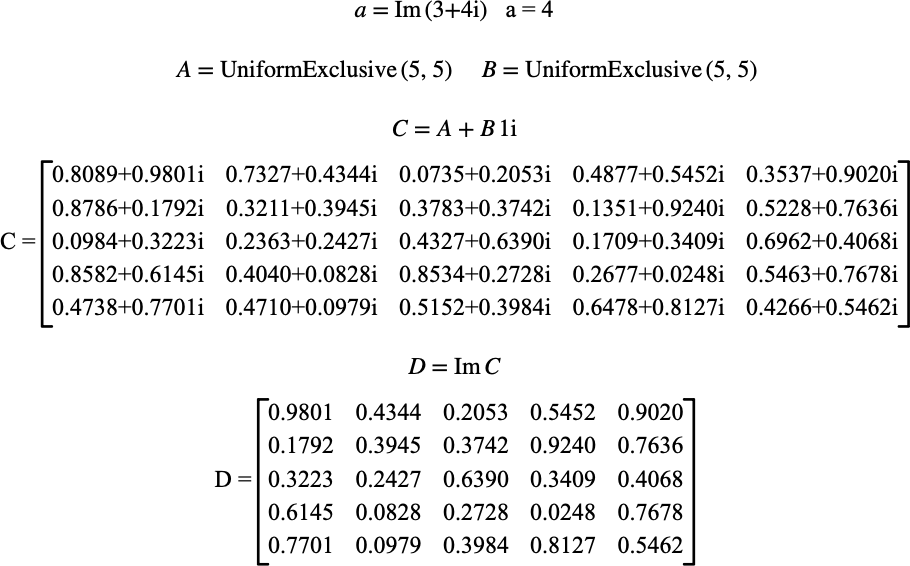# $$\text{Im}$$¶

You can use the $$\text{Im}$$ function to obtain the imaginary portion of a value. When applied to a matrix, this function will return a real matrix holding just the imaginary portion.

You can use the \im backslash command to insert this function.

The following variants of this function are available:

• $$\text{scalar } \text{Im} \left ( \text{<value>} \right )$$

• $$\text{matrix } \text{Im} \left ( \text{<matrix>} \right )$$

This function will return zero or false when applied to a boolean, integer, or real value. Similarly, if the supplied parameter is a boolean matrix, an integer matrix, or a real matrix, the function will return a zero matrix of the same type and size as the supplied matrix.

Figure 154 shows the basic use of the $$\text{Im}$$ function.Figure 154 Example Use Of The Im Function